# What Is Simple Harmonic Motion?

Many simple oscillators display the characteristic of Simple Harmonic Motion (SHM). Examples are springs, pendula, waves on the sea... all of which move in a predictable way and follow a certain pattern. Oscillators demonstrating SHM have displacement-time graphs that can be related to the graph of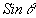or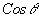plotted against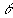, in other words regular Sine or Cosine curves.

Oscillators in simple harmonic motion follow several equations. These are related to different factors about the oscillator. For the purposes of this document I will confine these equations to springs, which can easily be used to test the ideas put forward.

An alternative derivation of SHM is also available, which uses a theoretical experiment to obtain by logical progression the proportionality contained in the equation.

From Hooke's Law we know that Force exerted by a spring on a mass attached to it is directly proportional to the extension of the spring, or: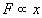Where F is the force exerted on the spring and x is the extension of the spring from its normal length. From this we can see that the equation relating these two vectors is: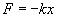Where k is Hooke's constant.

From this equation, and therefore from the slope of a Force against Displacement graph, we can calculate k.

With a mass with weight W Newtons attached but at rest, the spring stretches by distance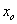. This then means that: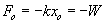as the force provided by the spring must be equal to the downward force provided by the mass.

If we pull the weight down a further distance x, the restoring force, F provided by the spring in an upwards direction acting on the mass is F+W. As at this position: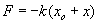Applying Newton's second Law of Motion, F=ma gives us: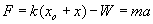As we know that from Hooke's Law for W the spring extends a certain distance: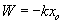We can then say: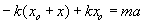Which can then be cancelled to give: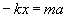orThis then relates a spring's Hooke's constant to the acceleration acting on a certain mass.

From one of the equations for SHM we can derive another equation: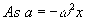comparing this with the above equation derived from F=ma: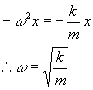As the period, T, of the motion of the mass on the spring is given by the general equation: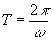we can substitute to give: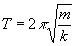where m is, in this case, the mass on the spring and k is the stiffness of the spring.